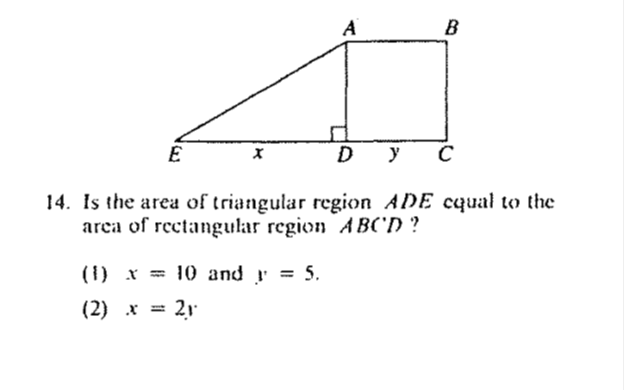# GMAT Quantitave Reasoning: Data Sufficiency Example Problem #3

“The GMAT Project” intends to ensure its readers are well prepared for all aspects of the GMAT. If you follow our weekly postings of example problems and solutions, we expect you to be more prepared than ever to not only succeed on the GMAT, but to get into your graduate school of choice.

This week we will turn our attention to a GMAT Data Sufficiency question. Because the instructions regarding Data Sufficiency questions are highly specific we have included them.

GMAT Data Sufficiency Instructions: Each of the data sufficiency questions below consist of a question and two statements, labeled (1) and (2), in which certain data are given. You have to decide whether the data given in the statements are ­sufficient for answering the question. Using the data given in the statement plus your knowledge of mathematics and everyday facts (such as the number of days in July or the meaning of counterclockwise) you are to fill in oval:

1. if statement (1) ALONE is sufficient, but statement (2) alone is not sufficient to answer the question asked;
2. if statement (2) ALONE is sufficient, but statement (1) alone is not sufficient to answer the question asked;
3. if BOTH statements (1) and (2) TOGETHER are sufficient to answer the question asked, but NEITHER statement ALONE is sufficient;
4. if EACH statement ALONE is sufficient to answer the question asked;
5. if statements (1) and (2) together are NOT sufficient to answer the question asked, and additional data specific to the problem are needed.Here is an example of the way that geometry concepts are tested in data sufficiency problems. It is important to have the necessary geometric formulas memorized before you take your exam.

This problem involves area. First, note that there is no way to determine the area of each region from the diagram. However, the question asks whether the two regions are equal. This means that we only have to show that the regions are definitely equal or definitely not equal.

Start with the formulas for area. The first region is a triangle. The area of a triangle is one half base times height.

AT = (1/2)bh

Note that the base of the triangle in the diagram is given as x. Substitute x in place of b.

AT = (1/2)xh

The second region is a rectangle. The area of a rectangle is base times height.

AR = bh

The base of the rectangle is y so substitute that in place of b.

AR = yh

Our two formulas then are AT = (1/2)xh and AR = yh. Note that the height of the triangle is equal to the height of the rectangle. This means that the variable h refers to the same value in both formulas.

1)      Plug in these values for x and y into the formulas.

AT = (1/2)xh

AT = (1/2)10h

AT = 5h

AR = yh

AR = 5h

Both areas are equal to 5h. Again, it isn’t important that we don’t know the actual value of the areas. We do know that both areas are equal. The first statement is sufficient.

2)      Since x is equal to 2y, substitute 2y in place of x in the first formula.

AT = (1/2)xh

AT = (1/2)2yh

AT = yh

Remember that the area of the rectangle is also equal to yh. Both areas are therefore equal. The second statement is sufficient.

Since both statements are sufficient on their own, the answer is (D).

By Robert Lynch